## Bucket Sort in Python

### Introduction

In this tutorial, we'll be diving into the theory and implementation of Bucket Sort in Python.

Bucket Sort is a comparison-type algorithm which assigns elements of a list we want to sort in Buckets, or Bins. The contents of these buckets are then sorted, typically with another algorithm. After sorting, the contents of the buckets are appended, forming a sorted collection.

Bucket Sort can be thought of as a scatter-order-gather approach towards sorting a list, due to the fact that the elements are first scattered in buckets, ordered within them, and finally gathered into a new, sorted list.

We'll implement Bucket Sort in Python and analyze it's time complexity.

### How does Bucket Sort Work?

Before jumping into its exact implementation, let's walk through the algorithm's steps:

1. Set up a list of empty buckets. A bucket is initialized for each element in the array.
2. Iterate through the bucket list and insert elements from the array. Where each element is inserted depends on the input list and the largest element of it. We can end up with 0..n elements in each bucket. This will be elaborated on in the visual presentation of the algorithm.
3. Sort each non-empty bucket. You can do this with any sorting algorithm. Since we're working with a small dataset, each bucket won't have many elements so Insertion Sort works wonders for us here.
4. Visit the buckets in order. Once the contents of each bucket are sorted, when concatenated, they will yield a list in which the elements are arranged based on your criteria.

Let's take a look at the visual presentation of how the algorithm works. For example, let's assume that this is the input list: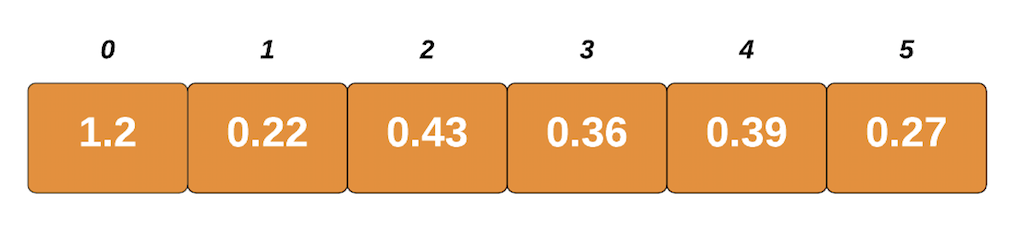The largest element is 1.2, and the length of the list is 6. Using these two, we'll figure out the optimal size of each bucket. We'll get this number by dividing the largest element with the length of the list. In our case, it's 1.2/6 which is 0.2.

By dividing the element's value with this size, we'll get an index for each element's respective bucket.

Now, we'll create empty buckets. We'll have the same amount of buckets as the elements in our list: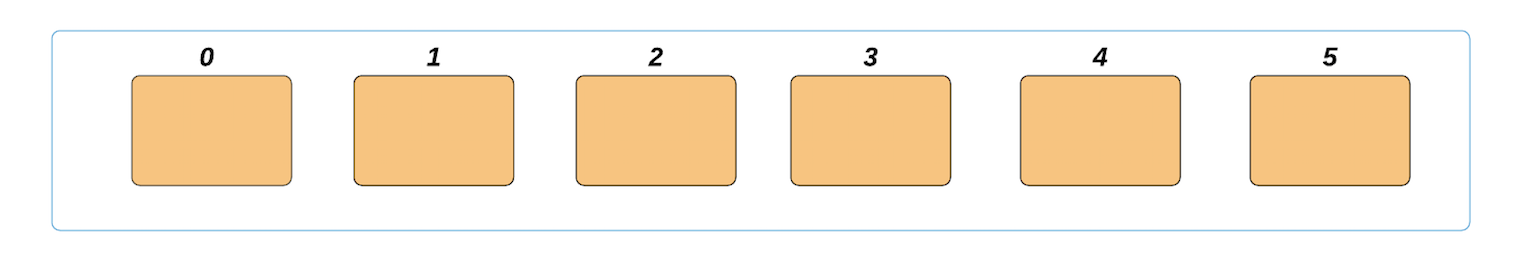We'll insert the elements in their respective buckets. Taking the first element into consideration - 1.2/0.2 = 6, the index of its respective bucket is 6. If this result is higher or equal to the length of the list, we'll just subtract 1 and it'll fit into the list nicely. This only happens with the largest number, since we got the size by dividing the largest element by the length.

We'll place this element in the bucket with the index of 5: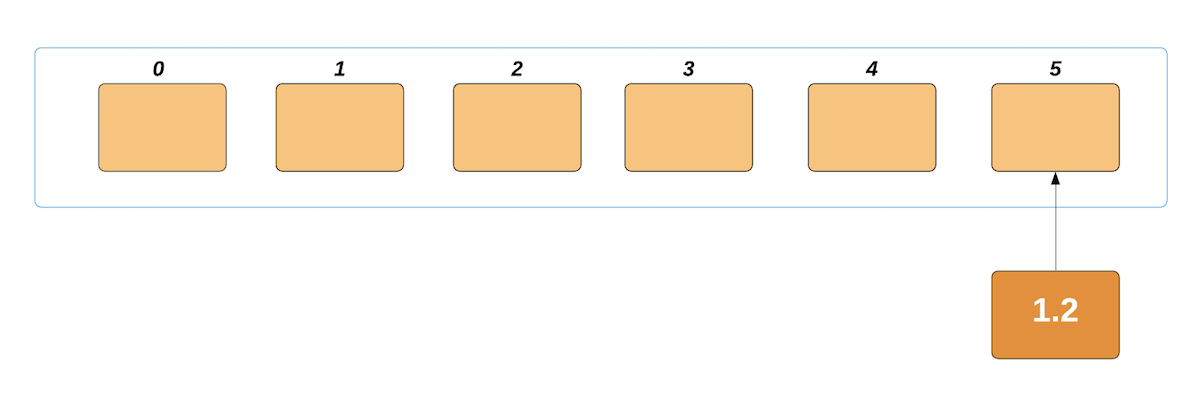Likewise, the next element will be indexed to 0.22/0.2 = 1.1. Since this is a decimal number, we'll floor it. This is rounded to 1, and our element is placed in the second bucket: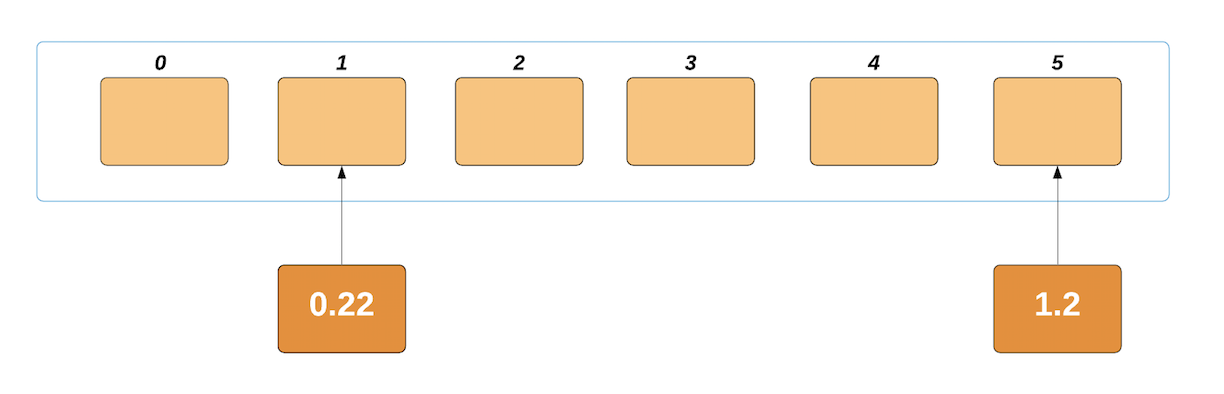This process is repeated until we've placed the last element in its respective bucket. Our buckets now look something along the lines of: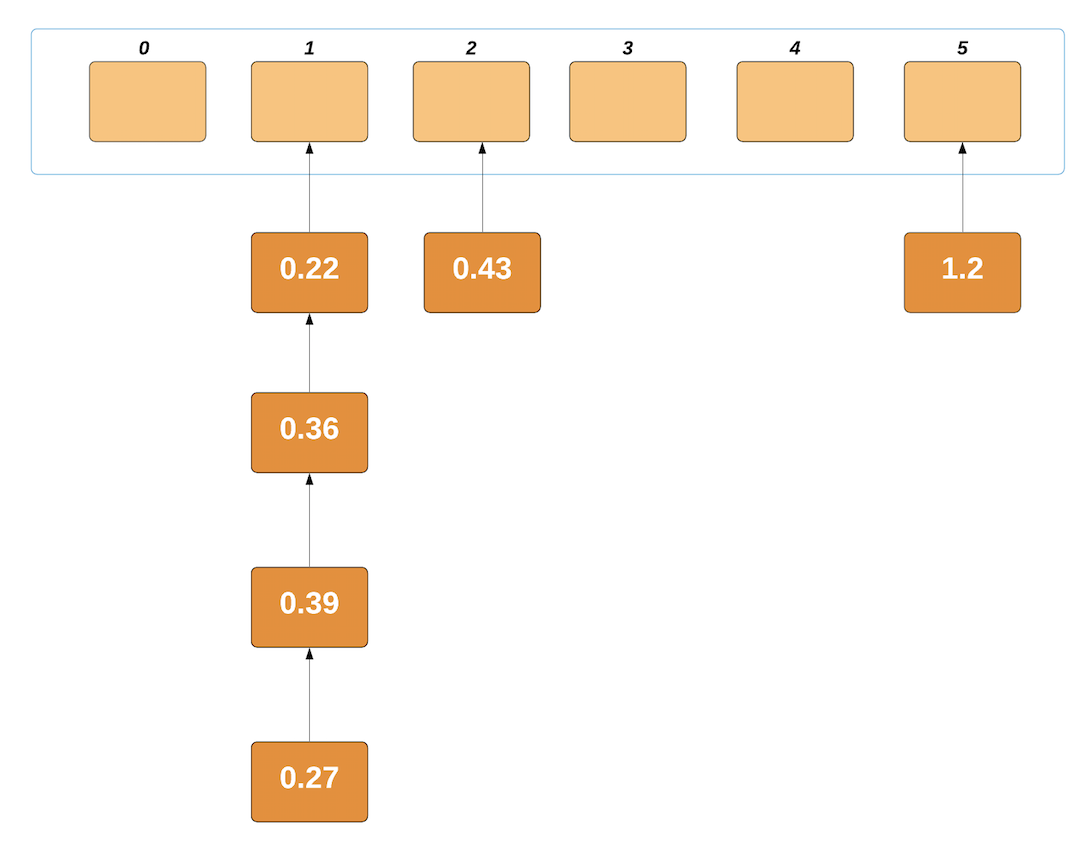Now, we'll sort the contents of each non-empty bucket. We'll be using Insertion Sort since it's unbeaten with small lists like this. After Insertion Sort, the buckets look like this: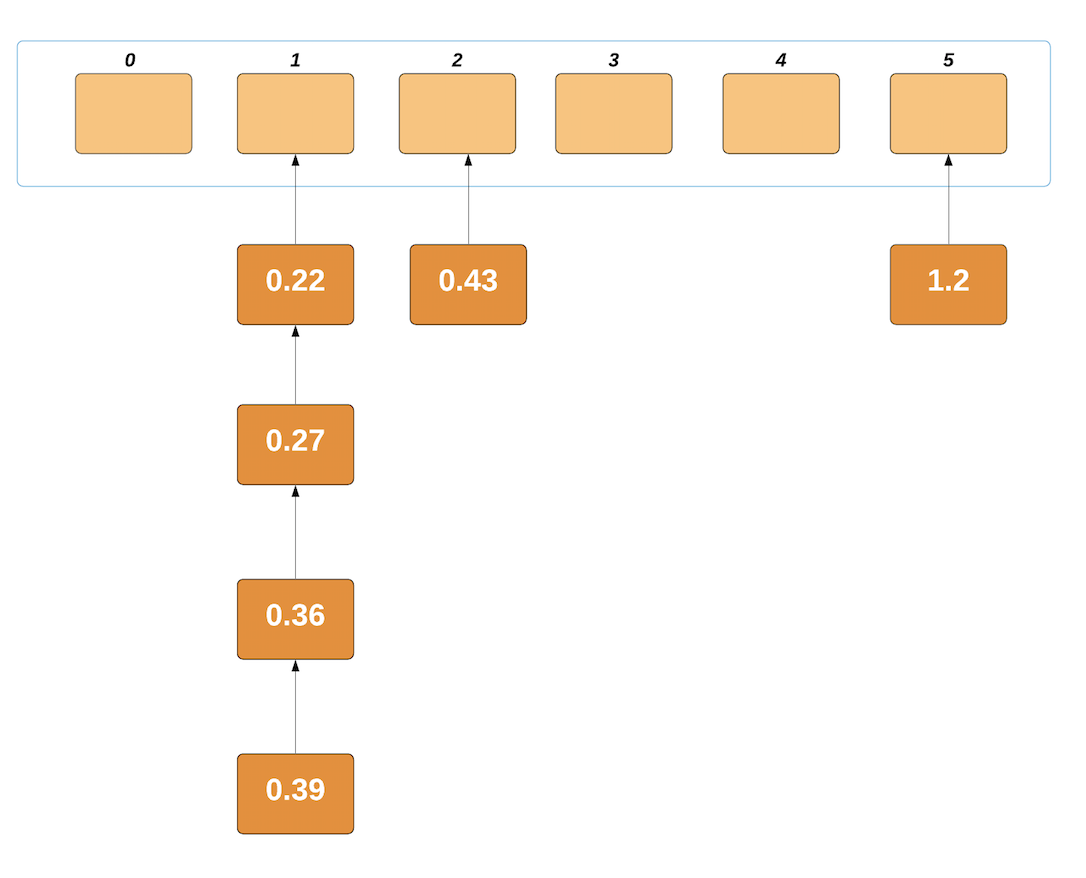Now, it's just a matter of traversing the non-empty buckets and concatenating the elements in a list. They're sorted and ready to go: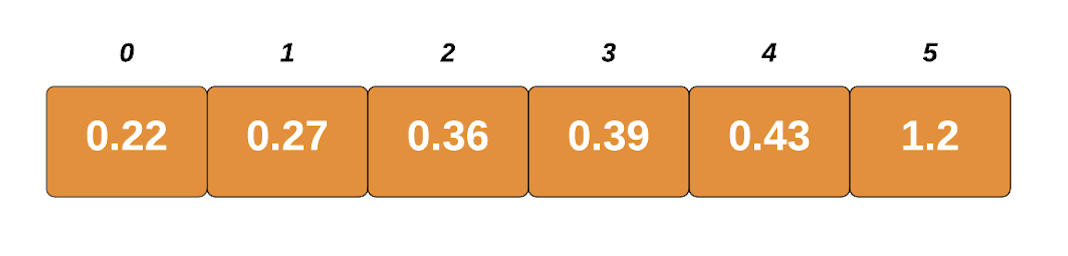### Bucket Sort Implementation in Python

With that out of the way, let's go ahead and implement the algorithm in Python. Let's start off with the bucket_sort() function itself:

def bucket_sort(input_list):
# Find maximum value in the list and use length of the list to determine which value in the list goes into which bucket
max_value = max(input_list)
size = max_value/len(input_list)

# Create n empty buckets where n is equal to the length of the input list
buckets_list= []
for x in range(len(input_list)):
buckets_list.append([])

# Put list elements into different buckets based on the size
for i in range(len(input_list)):
j = int (input_list[i] / size)
if j != len (input_list):
buckets_list[j].append(input_list[i])
else:
buckets_list[len(input_list) - 1].append(input_list[i])

# Sort elements within the buckets using Insertion Sort
for z in range(len(input_list)):
insertion_sort(buckets_list[z])

# Concatenate buckets with sorted elements into a single list
final_output = []
for x in range(len (input_list)):
final_output = final_output + buckets_list[x]
return final_output


The implementation is fairly straightforward. We've calculated the size parameter. Then, we instantiated a list of empty buckets and inserted elements based on their value and the size of each bucket.

Once inserted, we call insertion_sort() on each of the buckets:

def insertion_sort(bucket):
for i in range (1, len (bucket)):
var = bucket[i]
j = i - 1
while (j >= 0 and var < bucket[j]):
bucket[j + 1] = bucket[j]
j = j - 1
bucket[j + 1] = var


And with that in place, let's populate a list and perform a Bucket Sort on it:

def main():
input_list = [1.20, 0.22, 0.43, 0.36,0.39,0.27]
print('ORIGINAL LIST:')
print(input_list)
sorted_list = bucket_sort(input_list)
print('SORTED LIST:')
print(sorted_list)


Running this code will return:

Original list: [1.2, 0.22, 0.43, 0.36, 0.39, 0.27]
Sorted list: [0.22, 0.27, 0.36, 0.39, 0.43, 1.2]


### Bucket Sort Time Complexity

#### Worst-Case Complexity

If the collection we're working with has a short range (like the one we've had in our example) - it's common to have many elements in a single bucket, where a lot of buckets are empty.

If all elements fall into the same bucket, the complexity depends exclusively on the algorithm we use to sort the contents of the bucket itself.

Since we're using Insertion Sort - its worst-case complexity shines when the list is in reverse order. Thus, the worst-case complexity for Bucket Sort is also O(n2).

#### Best-Case Complexity

The best-case would be having all of the elements already sorted. Additionally, the elements are uniformly distributed. This means that each bucket would have the same number of elements.

That being said, creating the buckets would take O(n) and Insertion sort would take O(k), giving us an O(n+k) complexity.

#### Average-Case Complexity

The average-case occurs in the vast majority of real-life collections. When the collection we want to sort is random. In that case, Bucket Sort takes O(n) to finish, making it very efficient.

### Conclusion

To sum it all up, we started off by getting an introduction to what Bucket sort is and went on to discuss what we need to know before we jump into its implementation in Python. After the implementation, we've performed a quick complexity analysis.